# Building a Handwritten Digits Classifier¶

## 1) Introduction¶

In this project, we'll:

• explore why image classification is a hard task
• observe the limitations of traditional machine learning models for image classification
• train, test, and improve a few different deep neural networks for image classification

Deep neural networks have been used to reach state-of-the-art performance on image classification tasks in the last decade. For some image classification tasks, deep neural networks actually perform as well as or slightly better than the human benchmark. Some info about the history of deep neural networks can be read here.

In this project, we'll build models that can classify handwritten digits. Before the year 2000, institutions like the United States Post Office used handwriting recognition software to read addresses, zip codes, and more. One of their approaches, which consists of pre-processing handwritten images then feeding to a neural network model is detailed in this paper.

### Why is image classification a hard task?¶

Within the field of machine learning and pattern recognition, image classification (especially for handwritten text) is towards the difficult end of the spectrum. There are a few reasons for this.

First, each image in a training set is high dimensional. Each pixel in an image is a feature and a separate column. This means that a 128 x 128 image has 16384 features.

Second, images are often downsampled to lower resolutions and transformed to grayscale (no color). This is a limitation of compute power unfortunately. The resolution of a 8 megapixel photo has 3264 by 2448 pixels, for a total of 7,990,272 features (or about 8 million). Images of this resolution are usually scaled down to between 128 and 512 pixels in either direction for significantly faster processing. This often results in a loss of detail that's available for training and pattern matching.

Third, the features in an image don't have an obvious linear or nonlinear relationship that can be learned with a model like linear or logistic regression. In grayscale, each pixel is just represented as a brightness value ranging from 0 to 256.

Here's an example of how an image is represented across the different abstractions we care about: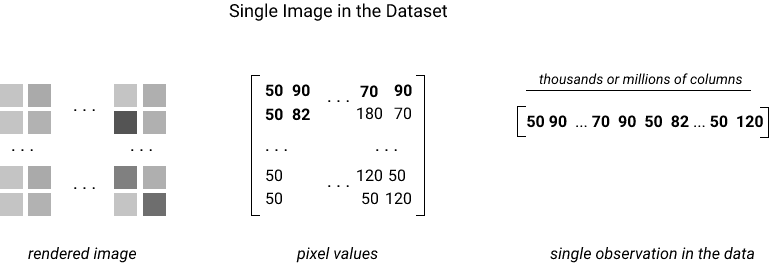### Why is deep learning effective in image classification?¶

Deep learning is effective in image classification because of the models' ability to learn hierarchical representations. At a high level, an effective deep learning model learns intermediate representations at each layer in the model and uses them in the prediction process. Here's a diagram that visualizes what the weights represent at each layer of a convolutional neural network, a type of network that's often used in image classification and unfortunately out of scope for this course, which was trained to identify faces.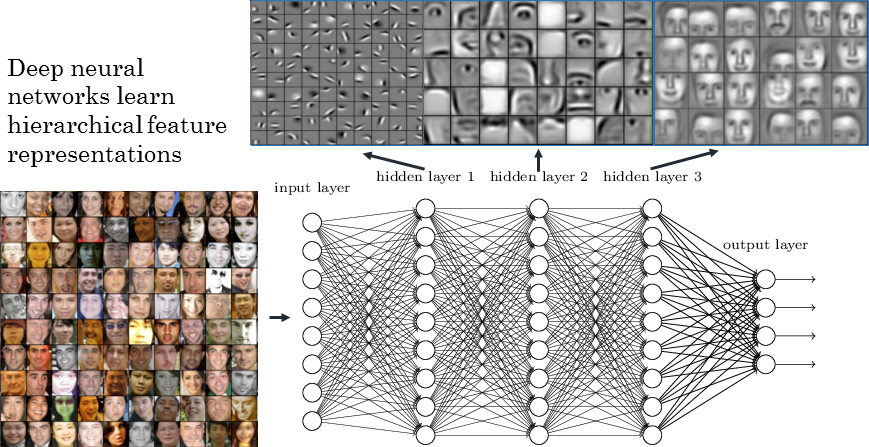The first hidden layer shows that the network learned to represent edges and specific features of faces. In the second hidden layer, the weights seemed to represent higher level facial features like eyes and noses. Finally, the weights in the last hidden layer resemble faces that could be matched against. Each successive layer uses weights from previous layers to try to learn more complex representations.

In this Guided Project, we'll explore the effectiveness of deep, feedforward neural networks at classifying images.

## 2) Data preparation¶

Scikit-learn contains a number of datasets pre-loaded with the library, within the namespace of sklearn.datasets. The load_digits() function returns a copy of the hand-written digits dataset from UCI.

Because dataframes are a tabular representation of data, each image is represented as a row of pixel values. To visualize an image from the dataframe, we need to reshape the image back to its original dimensions (28 x 28 pixels) and plot them on a coordinate grid.

### 2.1) Set up the environment¶

In :
import pandas as pd
import numpy as np
import matplotlib.pyplot as plt
from sklearn.neighbors import KNeighborsClassifier
from sklearn.metrics import accuracy_score
from sklearn.model_selection import KFold
from sklearn.neural_network import MLPClassifier


### 2.2) Import the data and initial inspection¶

In :
# Import the data as a tuple

# Convert the data to a Pandas dataframe, and append the last column
digits_df = pd.DataFrame(digits_tuple)
digits_df = pd.concat([digits_df, pd.DataFrame(digits_tuple)], axis=1)

# Display basic info
display(digits_df.info())

pixel_0_0 pixel_0_1 pixel_0_2 pixel_0_3 pixel_0_4 pixel_0_5 pixel_0_6 pixel_0_7 pixel_1_0 pixel_1_1 ... pixel_6_7 pixel_7_0 pixel_7_1 pixel_7_2 pixel_7_3 pixel_7_4 pixel_7_5 pixel_7_6 pixel_7_7 target
0 0.0 0.0 5.0 13.0 9.0 1.0 0.0 0.0 0.0 0.0 ... 0.0 0.0 0.0 6.0 13.0 10.0 0.0 0.0 0.0 0
1 0.0 0.0 0.0 12.0 13.0 5.0 0.0 0.0 0.0 0.0 ... 0.0 0.0 0.0 0.0 11.0 16.0 10.0 0.0 0.0 1
2 0.0 0.0 0.0 4.0 15.0 12.0 0.0 0.0 0.0 0.0 ... 0.0 0.0 0.0 0.0 3.0 11.0 16.0 9.0 0.0 2
3 0.0 0.0 7.0 15.0 13.0 1.0 0.0 0.0 0.0 8.0 ... 0.0 0.0 0.0 7.0 13.0 13.0 9.0 0.0 0.0 3
4 0.0 0.0 0.0 1.0 11.0 0.0 0.0 0.0 0.0 0.0 ... 0.0 0.0 0.0 0.0 2.0 16.0 4.0 0.0 0.0 4

5 rows × 65 columns

<class 'pandas.core.frame.DataFrame'>
RangeIndex: 1797 entries, 0 to 1796
Data columns (total 65 columns):
#   Column     Non-Null Count  Dtype
---  ------     --------------  -----
0   pixel_0_0  1797 non-null   float64
1   pixel_0_1  1797 non-null   float64
2   pixel_0_2  1797 non-null   float64
3   pixel_0_3  1797 non-null   float64
4   pixel_0_4  1797 non-null   float64
5   pixel_0_5  1797 non-null   float64
6   pixel_0_6  1797 non-null   float64
7   pixel_0_7  1797 non-null   float64
8   pixel_1_0  1797 non-null   float64
9   pixel_1_1  1797 non-null   float64
10  pixel_1_2  1797 non-null   float64
11  pixel_1_3  1797 non-null   float64
12  pixel_1_4  1797 non-null   float64
13  pixel_1_5  1797 non-null   float64
14  pixel_1_6  1797 non-null   float64
15  pixel_1_7  1797 non-null   float64
16  pixel_2_0  1797 non-null   float64
17  pixel_2_1  1797 non-null   float64
18  pixel_2_2  1797 non-null   float64
19  pixel_2_3  1797 non-null   float64
20  pixel_2_4  1797 non-null   float64
21  pixel_2_5  1797 non-null   float64
22  pixel_2_6  1797 non-null   float64
23  pixel_2_7  1797 non-null   float64
24  pixel_3_0  1797 non-null   float64
25  pixel_3_1  1797 non-null   float64
26  pixel_3_2  1797 non-null   float64
27  pixel_3_3  1797 non-null   float64
28  pixel_3_4  1797 non-null   float64
29  pixel_3_5  1797 non-null   float64
30  pixel_3_6  1797 non-null   float64
31  pixel_3_7  1797 non-null   float64
32  pixel_4_0  1797 non-null   float64
33  pixel_4_1  1797 non-null   float64
34  pixel_4_2  1797 non-null   float64
35  pixel_4_3  1797 non-null   float64
36  pixel_4_4  1797 non-null   float64
37  pixel_4_5  1797 non-null   float64
38  pixel_4_6  1797 non-null   float64
39  pixel_4_7  1797 non-null   float64
40  pixel_5_0  1797 non-null   float64
41  pixel_5_1  1797 non-null   float64
42  pixel_5_2  1797 non-null   float64
43  pixel_5_3  1797 non-null   float64
44  pixel_5_4  1797 non-null   float64
45  pixel_5_5  1797 non-null   float64
46  pixel_5_6  1797 non-null   float64
47  pixel_5_7  1797 non-null   float64
48  pixel_6_0  1797 non-null   float64
49  pixel_6_1  1797 non-null   float64
50  pixel_6_2  1797 non-null   float64
51  pixel_6_3  1797 non-null   float64
52  pixel_6_4  1797 non-null   float64
53  pixel_6_5  1797 non-null   float64
54  pixel_6_6  1797 non-null   float64
55  pixel_6_7  1797 non-null   float64
56  pixel_7_0  1797 non-null   float64
57  pixel_7_1  1797 non-null   float64
58  pixel_7_2  1797 non-null   float64
59  pixel_7_3  1797 non-null   float64
60  pixel_7_4  1797 non-null   float64
61  pixel_7_5  1797 non-null   float64
62  pixel_7_6  1797 non-null   float64
63  pixel_7_7  1797 non-null   float64
64  target     1797 non-null   int32
dtypes: float64(64), int32(1)
memory usage: 905.6 KB

None

The information above shows that our dataframe contains 1797 rows x 65 columns, with no null values at all.

Next, we'll inspect the first and the last 10 pictures.

In :
# Initiate a grid plot
print("First 10 images:")
plt.figure(figsize=(8,8))
# Iterate over the first 10 rows of the dataframe, excluding the "target" column, and display the image
for index, row in digits_df.iloc[:10, :-1].iterrows():
# Transform the row values to a Numpy array, then reshape it to a 8x8
reshaped_image = np.array(row).reshape(8,8)
# Create the subplot of the current row
plt.subplot(1, 10, index+1)
plt.imshow(reshaped_image, cmap='gray_r')
# Remove x and y axis for better visualization
ax = plt.gca()
ax.axes.xaxis.set_visible(False)
ax.axes.yaxis.set_visible(False)
# Plot the images
plt.show()

# Initiate a grid plot
print("Last 10 images:")
plt.figure(figsize=(8,8))
# Iterate over the last 10 rows of the dataframe, excluding the "target" column, and display the image
for index, row in digits_df.iloc[-10:, :-1].iterrows():
# Transform the row values to a Numpy array, then reshape it to a 8x8
reshaped_image = np.array(row).reshape(8,8)
# Create the subplot of the current row
plt.subplot(1, 10, index-digits_df.shape+11)
plt.imshow(reshaped_image, cmap='gray_r')
# Remove x and y axis for better visualization
ax = plt.gca()
ax.axes.xaxis.set_visible(False)
ax.axes.yaxis.set_visible(False)
# Plot the images
plt.show()

First 10 images: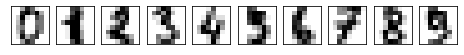Last 10 images: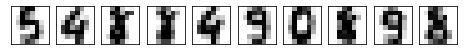## 3) Data analysis¶

First, we'll define some functions that will be useful across different machine learning models.

In :
def train(train_df, features, target, model):
"""
Fits a machine learning model on the train dataset

Args:
train_df: Training dataset
features: Predictors to be used
target: Target variable to predict
model: Machine learning model to use

Returns:
none
"""
model.fit(train_df[features], train_df[target])

def test(test_df, train_df, features, target, model):
"""
Tests a machine learning model on the test and train datasets

Args:
test_df: Test dataset
train_df: Training dataset
features: Predictors to be used
target: Target variable to predict
model: Machine learning model to use

Returns:
test_accuracy: Accuracy score for the given model on the test dataset
train_accuracy: Accuracy score for the given model on the train dataset
"""
test_predictions = model.predict(test_df[features])
test_accuracy = accuracy_score(test_df[target], test_predictions)

train_predictions = model.predict(train_df[features])
train_accuracy = accuracy_score(train_df[target], train_predictions)

return test_accuracy, train_accuracy

def cross_validate(df, features, target, model):
"""
Use a 4-folds cross validation model on a machine learning model

Args:
df: Original dataframe
features: Predictors to be used
target: Target variable to predict
model: Machine learning model to use

Returns:
average_accuracy: Average accuracy score over the 4 folds
"""
# Initiate empty lists to store test and train accuracy scores
test_accuracy_scores = []
train_accuracy_scores = []

kf = KFold(n_splits=4, shuffle=True, random_state=1)
# Split into train and test datasets after randomization, for each fold
for train_index, test_index in kf.split(df):
train_df = df.iloc[train_index].copy()
test_df = df.iloc[test_index].copy()

# Train the model
train(train_df, features, target, model)

# Make predictions
test_accuracy, train_accuracy = test(test_df, train_df, features, target, model)
test_accuracy_scores.append(test_accuracy)
train_accuracy_scores.append(train_accuracy)

test_average_accuracy = np.mean(test_accuracy_scores)
train_average_accuracy = np.mean(train_accuracy_scores)

return test_average_accuracy, train_average_accuracy


### 3.1) K-nearest neighbors¶

In :
# Initiate empty lists to store test and train accuracy scores
test_accuracy_scores = []
train_accuracy_scores = []

# Calculate accuracy of the k-nearest neighbors model using different k-values
k_values = range(1,10)
for k in k_values:
kn = KNeighborsClassifier(n_neighbors=k)
test_accuracy, train_accuracy = cross_validate(df=digits_df,
features=list(digits_df.columns[:-1]),
target="target",
model=kn)
test_accuracy_scores.append(test_accuracy)
train_accuracy_scores.append(train_accuracy)

# Plot the results
plt.plot(k_values, test_accuracy_scores)
plt.plot(k_values, train_accuracy_scores)
plt.legend(["test", "train"])
plt.xlabel("k value")
plt.ylabel("Accuracy score")
plt.show()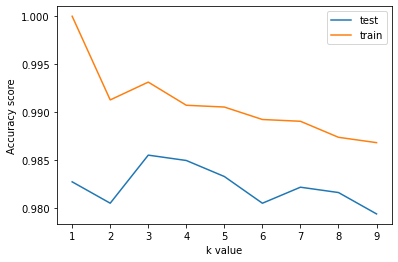The plot above shows that we have high accuracy scores with every k value that we've tested. The highest accuracy score (~98.6%) occurs with a k value of 3. We don't find much overfitting, as the accuracy scores are really high for the test dataset, leaving little room for overfitting on train accuracy scores.

However, there are a few downsides to using k-nearest neighbors:

• high memory usage (for each new unseen observation, many comparisons need to be made to seen observations)
• no model representation to debug and explore

Let's now try a neural network with a single hidden layer.

### 3.2) Neural network with a single hidden layer¶

In :
# Initiate empty lists to store test and train accuracy scores
test_accuracy_scores = []
train_accuracy_scores = []

# Calculate accuracy of the neural network model using different numbers of neurons
neurons = [8, 16, 32, 64, 128, 256]
for n in neurons:
mlp = MLPClassifier(hidden_layer_sizes=(n,))
test_accuracy, train_accuracy = cross_validate(df=digits_df,
features=list(digits_df.columns[:-1]),
target="target",
model=mlp)
test_accuracy_scores.append(test_accuracy)
train_accuracy_scores.append(train_accuracy)

# Plot the results
plt.plot(neurons, test_accuracy_scores)
plt.plot(neurons, train_accuracy_scores)
plt.legend(["test", "train"])
plt.xlabel("Number of neurons")
plt.ylabel("Accuracy score")
plt.show()

C:\Users\Alvaro\anaconda3\lib\site-packages\sklearn\neural_network\_multilayer_perceptron.py:614: ConvergenceWarning: Stochastic Optimizer: Maximum iterations (200) reached and the optimization hasn't converged yet.
warnings.warn(
C:\Users\Alvaro\anaconda3\lib\site-packages\sklearn\neural_network\_multilayer_perceptron.py:614: ConvergenceWarning: Stochastic Optimizer: Maximum iterations (200) reached and the optimization hasn't converged yet.
warnings.warn(
C:\Users\Alvaro\anaconda3\lib\site-packages\sklearn\neural_network\_multilayer_perceptron.py:614: ConvergenceWarning: Stochastic Optimizer: Maximum iterations (200) reached and the optimization hasn't converged yet.
warnings.warn(
C:\Users\Alvaro\anaconda3\lib\site-packages\sklearn\neural_network\_multilayer_perceptron.py:614: ConvergenceWarning: Stochastic Optimizer: Maximum iterations (200) reached and the optimization hasn't converged yet.
warnings.warn(
C:\Users\Alvaro\anaconda3\lib\site-packages\sklearn\neural_network\_multilayer_perceptron.py:614: ConvergenceWarning: Stochastic Optimizer: Maximum iterations (200) reached and the optimization hasn't converged yet.
warnings.warn(
C:\Users\Alvaro\anaconda3\lib\site-packages\sklearn\neural_network\_multilayer_perceptron.py:614: ConvergenceWarning: Stochastic Optimizer: Maximum iterations (200) reached and the optimization hasn't converged yet.
warnings.warn(
C:\Users\Alvaro\anaconda3\lib\site-packages\sklearn\neural_network\_multilayer_perceptron.py:614: ConvergenceWarning: Stochastic Optimizer: Maximum iterations (200) reached and the optimization hasn't converged yet.
warnings.warn(
C:\Users\Alvaro\anaconda3\lib\site-packages\sklearn\neural_network\_multilayer_perceptron.py:614: ConvergenceWarning: Stochastic Optimizer: Maximum iterations (200) reached and the optimization hasn't converged yet.
warnings.warn(
C:\Users\Alvaro\anaconda3\lib\site-packages\sklearn\neural_network\_multilayer_perceptron.py:614: ConvergenceWarning: Stochastic Optimizer: Maximum iterations (200) reached and the optimization hasn't converged yet.
warnings.warn(
C:\Users\Alvaro\anaconda3\lib\site-packages\sklearn\neural_network\_multilayer_perceptron.py:614: ConvergenceWarning: Stochastic Optimizer: Maximum iterations (200) reached and the optimization hasn't converged yet.
warnings.warn(
C:\Users\Alvaro\anaconda3\lib\site-packages\sklearn\neural_network\_multilayer_perceptron.py:614: ConvergenceWarning: Stochastic Optimizer: Maximum iterations (200) reached and the optimization hasn't converged yet.
warnings.warn(
C:\Users\Alvaro\anaconda3\lib\site-packages\sklearn\neural_network\_multilayer_perceptron.py:614: ConvergenceWarning: Stochastic Optimizer: Maximum iterations (200) reached and the optimization hasn't converged yet.
warnings.warn(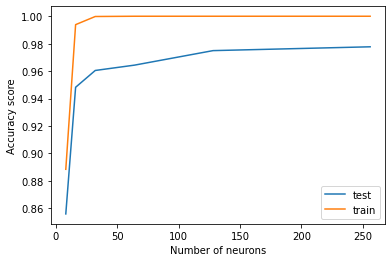The plot above shows that we have high accuracy scores with every number of neurons that we've tested. A very high accuracy score (~97%) occurs with 64 neurons, after which the accuracy score barely increases. We don't find much overfitting, as the accuracy scores are really high for the test dataset, leaving little room for overfitting on train accuracy scores.

Let's now try a neural network with two hidden layers.

### 3.3) Neural network with two hidden layers¶

In :
# Calculate accuracy of the neural network model using two layers of 64 neurons
mlp = MLPClassifier(hidden_layer_sizes=(64, 64))
test_accuracy, train_accuracy = cross_validate(df=digits_df,
features=list(digits_df.columns[:-1]),
target="target",
model=mlp)

# Plot the results
plt.scatter("two layers", test_accuracy)
plt.scatter("two layers", train_accuracy)
plt.legend(["test", "train"])
plt.ylabel("Accuracy score")
plt.show()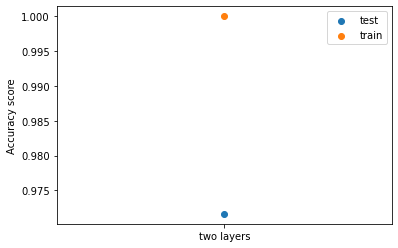The plot above shows that we have a high accuracy score (~97%) with 64 neurons on each of the two layers. We don't find much overfitting, as the accuracy score is really high for the test dataset, leaving little room for overfitting on train accuracy score.

Let's now try a neural network with three hidden layers and different number of neurons.

### 3.4) Neural network with three hidden layers¶

In :
# Initiate empty lists to store test and train accuracy scores
test_accuracy_scores = []
train_accuracy_scores = []

# Calculate accuracy of the neural network model using different numbers of neurons
neurons = [10, 64, 128]
for n in neurons:
mlp = MLPClassifier(hidden_layer_sizes=(n, n, n))
test_accuracy, train_accuracy = cross_validate(df=digits_df,
features=list(digits_df.columns[:-1]),
target="target",
model=mlp)
test_accuracy_scores.append(test_accuracy)
train_accuracy_scores.append(train_accuracy)

# Plot the results
plt.plot(neurons, test_accuracy_scores)
plt.plot(neurons, train_accuracy_scores)
plt.legend(["test", "train"])
plt.xlabel("Number of neurons")
plt.ylabel("Accuracy score")
plt.show()

C:\Users\Alvaro\anaconda3\lib\site-packages\sklearn\neural_network\_multilayer_perceptron.py:614: ConvergenceWarning: Stochastic Optimizer: Maximum iterations (200) reached and the optimization hasn't converged yet.
warnings.warn(
C:\Users\Alvaro\anaconda3\lib\site-packages\sklearn\neural_network\_multilayer_perceptron.py:614: ConvergenceWarning: Stochastic Optimizer: Maximum iterations (200) reached and the optimization hasn't converged yet.
warnings.warn(
C:\Users\Alvaro\anaconda3\lib\site-packages\sklearn\neural_network\_multilayer_perceptron.py:614: ConvergenceWarning: Stochastic Optimizer: Maximum iterations (200) reached and the optimization hasn't converged yet.
warnings.warn(
C:\Users\Alvaro\anaconda3\lib\site-packages\sklearn\neural_network\_multilayer_perceptron.py:614: ConvergenceWarning: Stochastic Optimizer: Maximum iterations (200) reached and the optimization hasn't converged yet.
warnings.warn(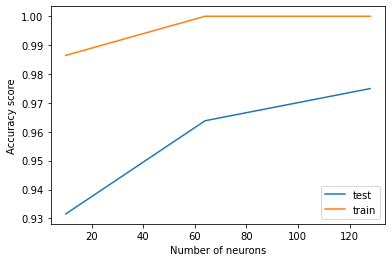Again, The plot above shows that we reached high accuracy scores for each test we run. There is a very high accuracy score (~97%) with 64 neurons on each of the three layers, after which the accuracy score barely increases. We don't find much overfitting, as the accuracy scores are really high for the test dataset, leaving little room for overfitting on train accuracy scores.

## 4) Conclusion¶

We can conclude that every single model that we've tested on this project works nice for classifying the images that we've been given.